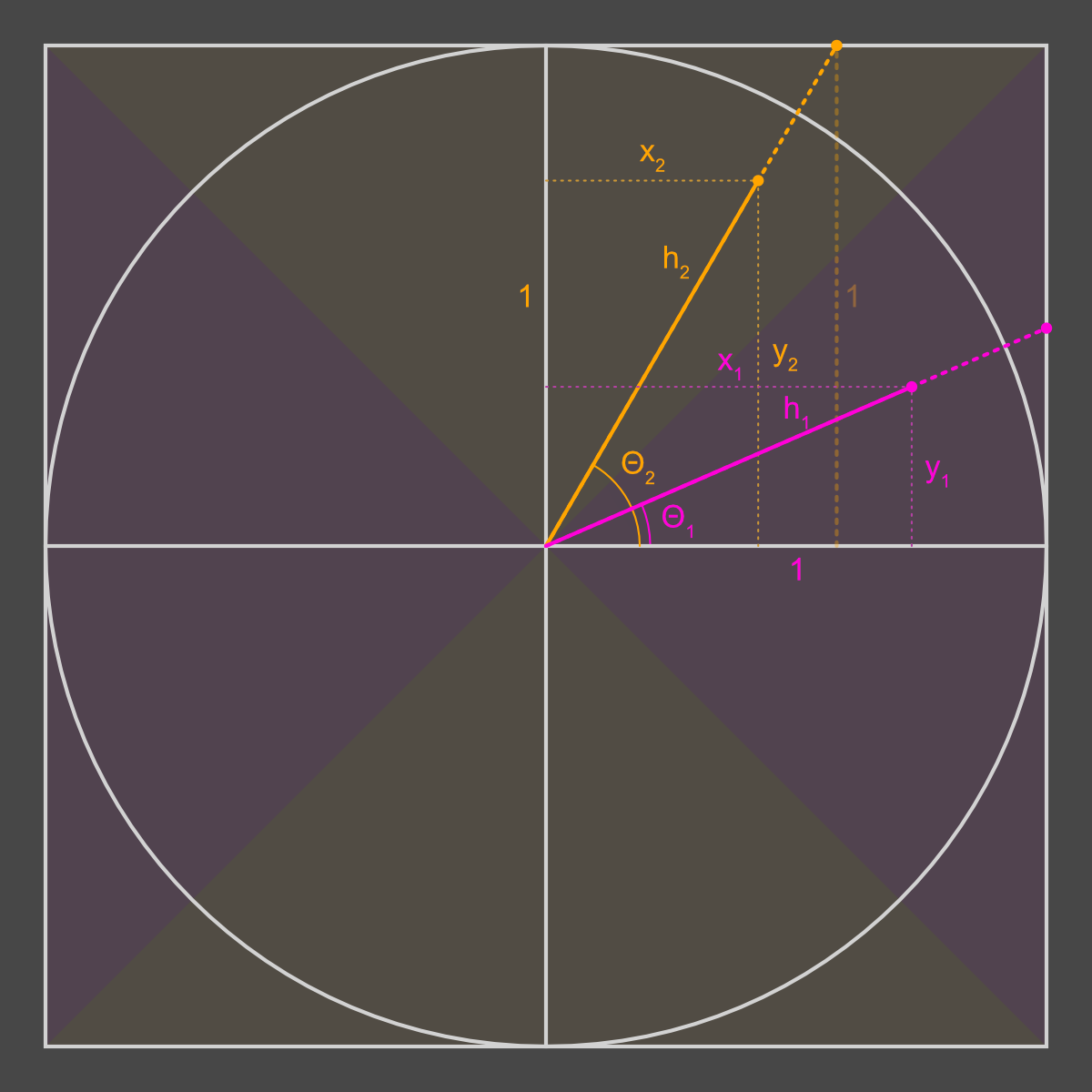# Joysticks

A while back, I wrote an answer to a forum question on how to calculate the overall intensity (magnitude) of a joystick axis... 6 years after the question was asked. Yeah, I'm one of those, sorry. This is a copy of that post for my own records, in case that answer ever goes away. Hopefully it's helpful to you as well!

I decided to answer this question because I was looking for the answer myself, not for a Unity project but for a project in JS. I figured the Unity forums would be a pretty good knowledge source for joystick-related issues, so I happily clicked that search result. Unfortunately, the two existing answers (including the accepted one) were wrong. Worse still, when I read them, I knew exactly what those felt like in videogames (games where your fastest movement speed is when you are moving at a 45° angle). Anyways, here's my answer:

In order to do this right, you have to normalise against the maximum possible magnitude for that angle. If you just clamp the magnitude of the vector to `[0, 1]`, then you only have to move `Mathf.Sqrt(magnitude)` in any diagonal direction to get the same normalised magnitude as moving `magnitude` in a horizontal or vertical direction. You run into the opposite problem if you just take the max of the 2 magnitudes, where diagonals are underrepresented instead of overrepresented.

## The code (C#, using Unity's `Vector2` and `Mathf`)

```    Vector2 vector = new Vector2(
Input.GetAxis("Horizontal"),
Input.GetAxis("Vertical")
);

float angle = Mathf.Atan2(vector.x, vector.y);
float maxMagnitude = Mathf.Abs(vector.x) > Mathf.Abs(vector.y)
? 1 / Mathf.Sin(angle)
: 1 / Mathf.Cos(angle);
float magnitude = abs(vector.magnitude / maxMagnitude);
```

The code above is C# and Unity specific, but it's pretty easy to adapt for any environment/language that has basic floating-point or decimal math functions and where the joystick input is 2 separate axes, each in the range of `[-1, 1]`. Since I was looking for a JS answer, it would be rude of me not to include my JS solution:

```    function vectorFromJoystick({ x, y }) {
const direction = Math.atan2(y, x);
const directionAbsolute = direction < 0
? TAU + direction
: direction;

const rawMagnitude = Math.sqrt(x ** 2 + y ** 2);
const maxMagnitude = Math.abs(x) > Math.abs(y)
? 1 / Math.sin(direction)
: 1 / Math.cos(direction);
const magnitude = rawMagnitude / Math.abs(maxMagnitude);

return { direction, directionAbsolute, magnitude };
}
```

I had some additional requirements/concerns with this that came out of how JS's built-in `Math` functions work. `Math.atan2` returns a number in the range `[-π, π]`. I added `directionAbsolute` which converts that to its `[0, 2π]` unit circle equivalent. That might be an issue with the C# code as well, as I did not test that as heavily as the JS one.

## The explanationThe circle here is the unit circle, and the enclosing square encloses all possible {x,y} values that can be given back by a joystick. The orange and pink dots are 2 example positions of the joystick, which fall into the 2 separate pink and orange tinted parts of the graph respectively. These tinted areas represent the 2 different cases that are handled by the ternary in the code above — more on that later.

The fundamental problem is that the magnitude coming out of the vector is relative to that outer square, but you want the magnitude relative to the unit circle, so that it is always in the range [0, 1]. You also want it to stay proportional no matter where the joystick is, so if the joystick's current values fall outside of the unit circle (e.g. `{ x: 0.9, y: 0.9 }`) the output magnitude should be almost but not quite 100%, because the user isn't actually pushing the joystick full-speed-ahead in that direction.

The solution is pretty straightforward: we take the vector's value and divide it by the maximum possible magnitude at that angle. In the diagram, the vector's value is the solid coloured line, and the maximum possible magnitude is that plus the dotted line extending all the way to the edge of the square.

Like I said before, there are 2 cases. Both are gonna use some trig to calculate the maximum possible lengths, taking advantage of the fact that we're working with right triangles.

### The pink case

Looking at the diagram, we know θ1 (the `angle`) and the length of the adjacent side from that corner (that pink 1). We want to find h1, which is the maximum possible magnitude at that angle. Referring to your trigonometric ratios (or wikipedia if you're like me and forgot them all) you'll see that the cosine of an angle θ is the ratio between the adjacent side and the hypotenuse, so we can use that to calculate the length of the hypotenuse. The formula is:

```    cos(θ1) = adjacent / hypotenuse = 1 / h1
```
Solving for h1, we get:
```    h1 = 1 / cos(θ1)
```

### The orange case

The problem we're solving is exactly the same here: how long is the hypotenuse (`h2` in this case). However, the 1-length side is now opposite θ2 instead of adjacent to it (you can see this side in faint dotted orange). No problem, as sine is the ratio between the opposite and hypotenuse.

```    sin(θ2) = opposite / hypotenuse = 1 / h2
```
again, solving for h2 this time:
```    h2 = 1 / sin(θ2)
```